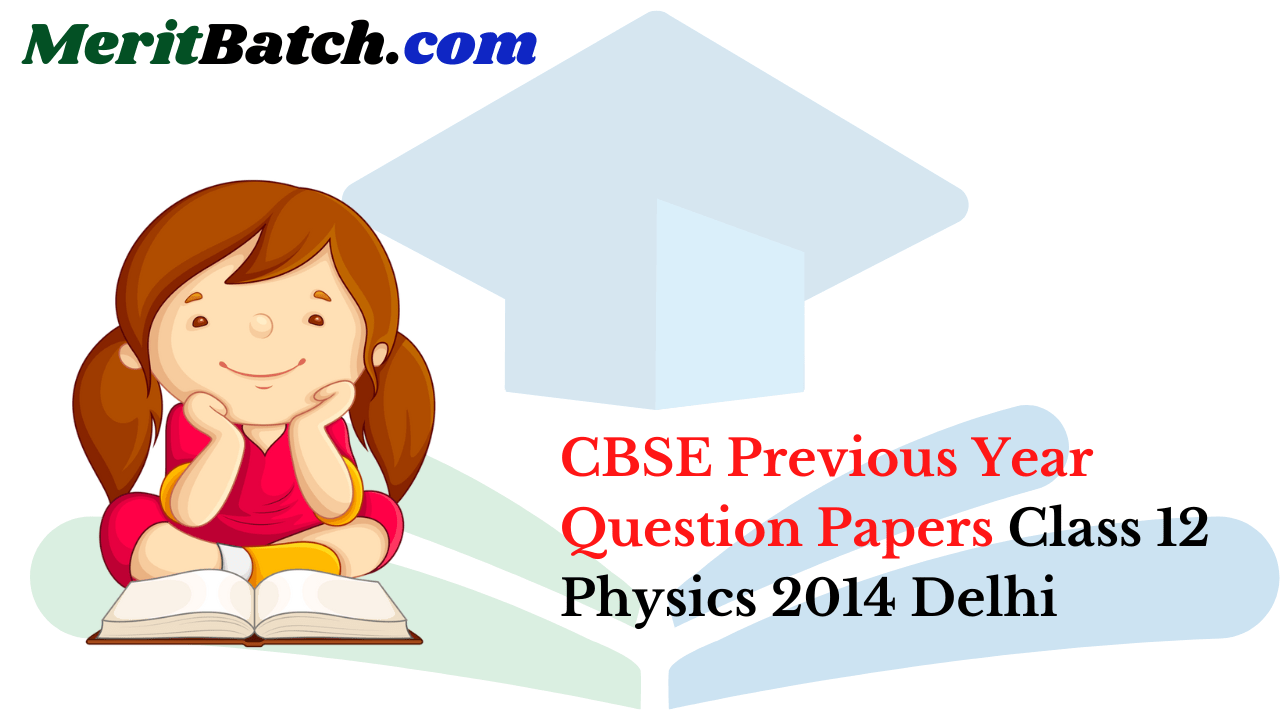## CBSE Previous Year Question Papers Class 12 Physics 2014 Delhi

### CBSE Previous Year Question Papers Class 12 Physics 2014 Delhi Set-I

Question 1.
Define the term ‘mobility’ of charge carriers in a conductor, write its S.I. unit. 
Mobility of charge carriers in a conductor is defined as the magnitude of their drift velocity per unit applied electric field.
Mobility, μ = Drift of electric field
$$\mu=\frac{v_{d}}{\mathrm{E}}$$
S.I. unit of mobility is m2V-1 s-1.

Question 2.
The carrier wave is given by C(t) = 2 sin (8πt) volt.
The modulating signal is a square wave as shown. Find modulation index.Question 3.
For any charge configuration, equipotential surface through a point is normal to the electric field. Justify. 
If the electric field were not normal ‘ to equipotential surface, it would have non zero component along the surface. To move a charge against this component, work would have to be done. But no work is needed to move a test charge on an equipotential surface. Hence electric field must be normal to the equipotential Surface at every point.

Question 4.
Two spherical bobs, one metallic and the other of glass, of the same size are allowed to fall freely from the same height above the ground. Which of the two would reach earlier and why ? 
Glass bob will reach the ground earlier than the metallic bob. As the metallic bob falls, it intercepts earth’s magnetic field and induced currents are set up in it which oppose its downward motion. But no such currents are induced in the glass bob.

Question 5.
Show variation of resistivity of copper as a function of temperature in a graph. 
The variation of resistivity of copper with temperature is parabolic in nature. This is shown in the following graph :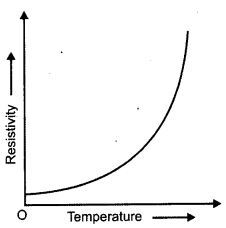Question 6.
A convex lens is placed in contact with a plane mirror. A point object at a distance of 20 cm on the axis of this combination has its image coinciding with it self. What is the focal length of the lens ? 
The figure shows a convex lens L placed in contact with a plane mirror M. P is the point object, kept in front of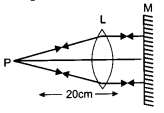this combination at a distance of 20 cm, from it. As the image coincides with itself, the rays from the object, after refraction from lens, should fall normally on the mirror M, so that they retrace their path. For this, the rays from P, after refraction from the lens must form a parallel beam perpendicular to M. For clarity, M has been shown at a small distance from L (in diagram). As the rays from P, form a parallel beam after refraction, P must be at the focus of the lens. Hence, the focal length of the lens is 20 cm.

Question 7.
Write the expression, in a vector form, for the Lorentz magnetic force $$\overrightarrow{\mathbf{F}}$$ due to a charge moving with velocity $$\overrightarrow{\mathbf{v}}$$ in a magnetic field $$\overrightarrow{\mathbf{B}}$$. What is the direction of the magnetic force ? 
The Lorentz magnetic force is given by the following relation:
$$\overrightarrow{\mathrm{F}}=\mathrm{q}(\vec{v} \times \overrightarrow{\mathrm{B}})$$

Here q, is the magnitude of the moving charge. The direction of the magnetic force is perpendicular to the plane containing the
velocity vector$$\overrightarrow{\mathbf{v}}$$ and the magnetic field vector $$\overrightarrow{\mathbf{B}}$$.

Question 8.
The figure given below shows the block diagram of a generalized communication system. Identify the element labelled ‘X’ and write its function.Question 9.
Out of the two magnetic materials, ‘A’ has relative permeability slightly greater than unity while ‘B’ has less than unity. Identify the nature of the materials ‘A’ and ‘B’. Will their susceptibilities be positive or negative ? 
For a para magnetic material, the relative permeability lies between 1 < µr < 1 + ∈ and its susceptibility lies between 0 < x < ∈.

Hence, ‘A’ is a para magnetic material and its susceptibility is positive. This is because its relative permeability is slightly greater than unity. For a diamagnetic material, the relative permeability lies between 0 ≤ µr < 1 and its susceptibility lies between -1 < x < 0. Hence, ‘B’ is a diamagnetic material and its susceptibility is negative. This is because its relative permeability is less than unity. Here µr and x refer to the relative permeability and susceptibility.

Question 10.
Given a uniform electric field $$\overset { \rightarrow }{ E } =5\times { 10 }^{ 3 }\overset { \wedge }{ i } N/c$$ find the flux of this field through a square of 10 cm on a side whose plane is parallel to the YZ plane. What would be the flux through the same square if the plane makes a 30° angle with the X-axis ? 
When the plane is parallel to the Y-Z plane: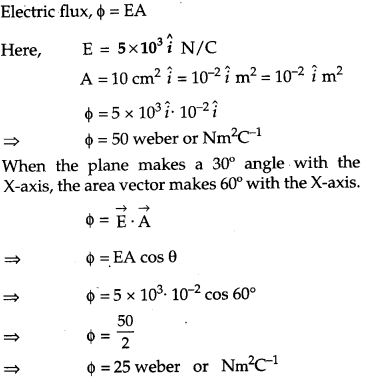Question 11.
For a single slit of width a, the first minimum of the interference pattern of a monochromatic light of wavelength λ occurs at an angle of λ /a At the same angle of λ /a we get a maximum for two a narrow slits separated by a distance a. Explain. 
When a single slit is used, the interference pattern is due to the diffraction phenomenon. In case of diffraction from a single slit of width a using monochromatic light of wavelength λ, the first minimum of the interference pattern occurs at an angle θ, which is given by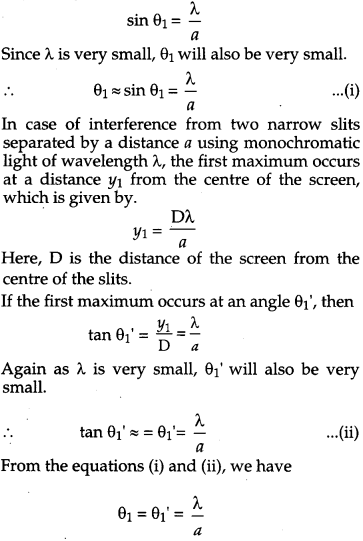Hence, it proves the result.

Question 12.
Write the truth table for the combination of the gates shown. Name the gates used.Identify the logic gates marked ‘P’ and ‘Q’ in the given circuit. Write the truth table for the combination.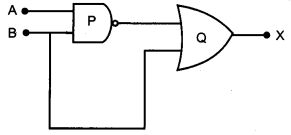Question 13.
State Kirchhoff’s rules. Explain briefly how these rules are justified. 
Kirchhoff’s first Law-Junction Rule In an electrical circuit, the algebraic sum of the currents meeting at a junction is always zero.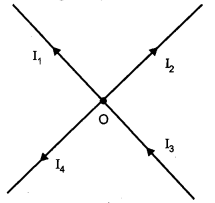I1, I2,13 and I4 are the currents flowing through the respective wires.
Convention : The current flowing towards the junction is taken as positive. The current flowing away from the junction is taken as negative.
I3 + (-I1) + (-I2) + (-I4) = 0
This law is based on the law of conservation of charge.

Kirchhoff’s Second Law – Loop rule
In a closed loop, the algebraic sum of the emfs is equal to the algebraic sum of the products of the resistances and the currents flowing through them.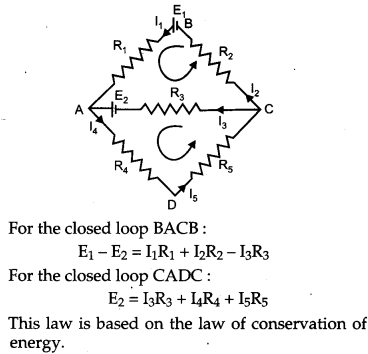Question 14.
A capacitor ‘C’, a variable resistor ‘R’ and a bulb ‘B’ are connected in series to the ac mains in circuit as shown. The bulb glows with some brightness. How will the glow of the bulb change if
(i) a dielectric slab is introduced between the plates of the capacitor, keeping resistance R to be the same;
(ii) the resistance R is increased keeping the same capacitance ?(i) As the dielectric slab is introduced between the plates of the capacitor, its capacitance will increase. Hence, the potential drop across the capacitor will decrease (V= Q/C). As a result, the potential drop across the bulb will increase (since both are connected in series). So, its brightness will increase.
(ii) As the resistance (R) is increased, the potential drop across the resistor will increase. As a result, the potential drop across the bulb will decrease (since both are connected in series). So, its brightness will decrease.

Question 15.
State the underlying principle of a cyclotron. Write briefly how this machine is used to accelerate charged particles to high energies. 
The underlying principle of a cyclotron is that an oscillating electric field can be used to accelerate a charged particle to high energy.

A cyclotron involves the use of an electric field to accelerate charge particles across the gap between the two D-shaped magnetic field regions. The magnetic field is perpendicular to the paths of the charged particles that makes them follow in circular paths within the two Ds. An alternating voltage accelerates the charged particles each time they cross the Ds. The radius of each particle’s path increases with its speed. So, the accelerated particles spiral toward the outer wall of the cyclotron.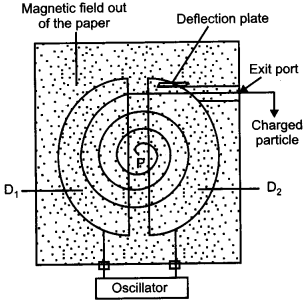The Ds are the semi-circular structures (D1 and D2) between which the charges move. The accelerating voltage is maintained across the opposite halves of the Ds. Square wave electric fields are used to accelerate the charged particles in a cyclotron.The accelerating electric field reverses just at the time the change particle finishes its half circle. So that it gets accelerated across the gap between the Ds.

The particle gets accelerated again and again, and its velocity increases. Therefore, it attains high kinetic energy. The positively charged ion adopts a circular path with a constant speed v, under the action of magnetic field B, which is perpendicular to the planes of D’s of radius r.
$$r=\frac{m v}{q \mathrm{B}}$$

Question 16.
An electric dipole of length 4 cm, when placed with its axis making an angle of 60° with a uniform electric field, experiences a torque of 4√3 Nm. Calculate the potential energy of the dipole, if it has charge ± 8 nC.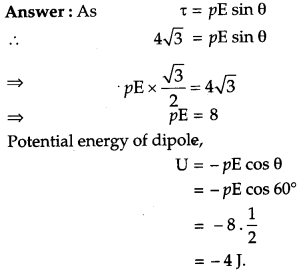Question 17.
A proton and a deuteron are accelerated through the same accelerating potential. Which one of the two has
(a) greater value of de-Broglie wavelength associated with it, and
(b) less momentum ?
(a) de-Broglie wavelength, λ ∝ 1 /mass (for same accelerating potential) Mass of a proton is less as compared to a deuteron. So, proton will have greater value of de-Broglie wavelength associated with it.
(b) Momentum, p ∝ mass (for same accelerating potential) Mass of deuteron is more as compared to a proton. So, it will have a greater value of momentum.

Question 18.
(i) Monochromatic light of frequency 6.0 x 1014 Hz is produced by a laser. The power emitted is 2.0 x 10-3 W. Estimate the number of photons emitted per second on an average by the source.
(ii) Draw a plot showing the variation of photoelectric current versus the intensity of incident radiation on a given photosensitive surface.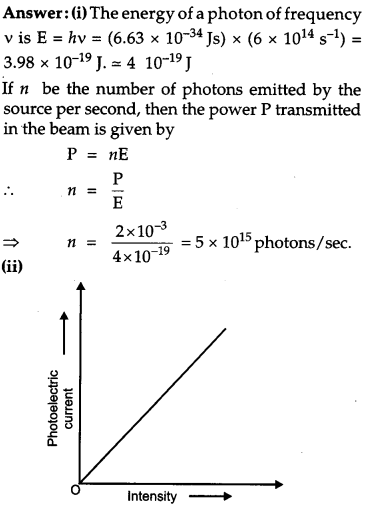Question 19.
A 12.5 eV electron beam is used to bombard gaseous hydrogen at room temperature. Up to which energy level the hydrogen atoms would be excited ? Calculate the wavelength of the first member of Lyman and first member of Balmer series.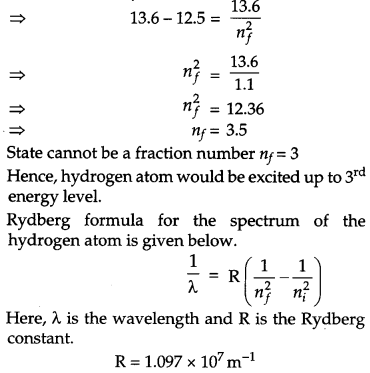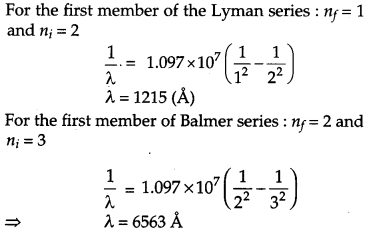Question 20.
When Sunita, a class XII student, came to know that her parents are planning to rent out the top floor of their house to a mobile company she protested. She tried hard to convince her parents that this move would be a health hazard. Ultimately her parents agreed : 
(1) In what way can the setting up of transmission tower by a mobile company in a residential colony prove to be injurious to health ?
(2) By objecting to this move of her parents, what value did Sunita display ?
(3) Estimate the range of e.m. waves which can be transmitted by an antenna of height 20 m. (Given radius of the earth = 6400 km) 
(1) A transmitting tower makes use of electromagnetic waves such as microwaves, exposure to which can cause severe health hazards like, giddiness, headache, tumour and cancer. Also, the transmitting antenna operates on a very high power, so the risk of someone getting severely burnt in a residential area increases.
(3) Range of the transmitting antenna.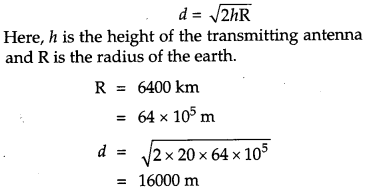Question 21.
A potentiometer wire of length 1 m has a resistance of 10 Ω. It is connected to a 6 V battery in series with a resistance of 5 Ω. Determine the emf of the primary cell which gives a balance point at 40 cm. 
From the figure below :Question 22.
(a) Draw a labelled ray diagram showing the formation of a final image by a compound microscope at least distance of distinct vision.
(b) The total magnification produced by a compound microscope is 20. The magnification produced by the eye piece is 5. The microscope is focused on a certain object. The distance between the objective and eye-piece is observed to be 14 cm. If least distance of distinct vision is 20 cm, calculate the focal length of the objective and the eye-piece. 
(a)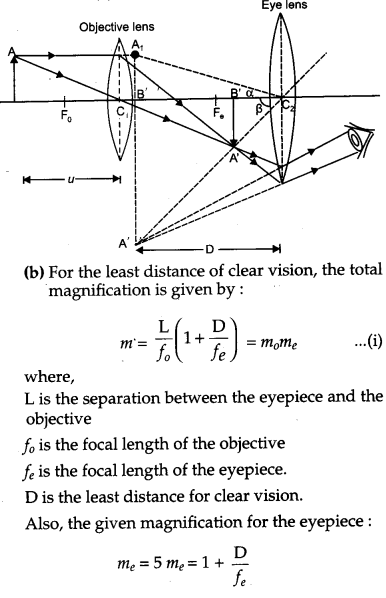Question 23.
(a) A mobile phone lies along the principal axis of a concave mirror. Show, with the help of a suitable diagram, the formation of its image. Explain why magnification is not uniform.
(b) Suppose the lower half of the concave mirror’s reflecting surface is covered with an opaque material. What effect this will have on the image of the object ? Explain. 
(a) The image of the mobile phone formed by the convave mirror will be as shown in fig. given below :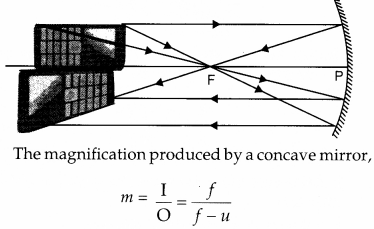Since the object distance for the different parts of the mobile phone along its length are different, the different parts will be magnified differently. Hence, the magnification is not uniform.
(b) As the laws of reflection are true for all points of the mirror, the height of the whole image will be produced. However, as the area of the reflecting surface has been reduced, the image intensity will be reduced. In other words, the image produced will be less bright.

Question 24.
(a) Obtain the expression for the energy stored per unit volume in a charged parallel plate capacitor.
(b) The electric field inside a parallel plate capacitor is E. Find the amount of work done in moving a charge q over a closed rectangular loop abcda.OR
(a) Derive the expression for the capacitance of a parallel plate capacitor having plate area A and plate separation d.
(b) Two charged spherical conductors of radii R1 and R2 when connected by a conducting wire acquire charge q1 and q2 respectively. Find the ratio of their surface charge densities in terms of their radii.
(a) Let us consider a parallel-plate capacitor of plate area A. If separation between plates is d metre, capacitance C is given by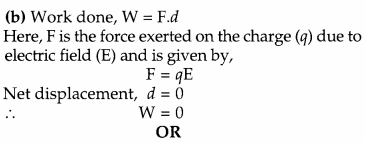(a) Derivation for the capacitance of parallel plate capacitor: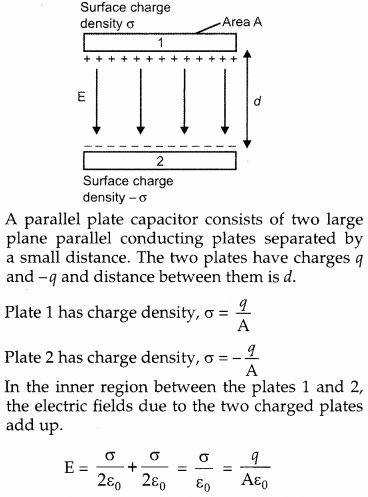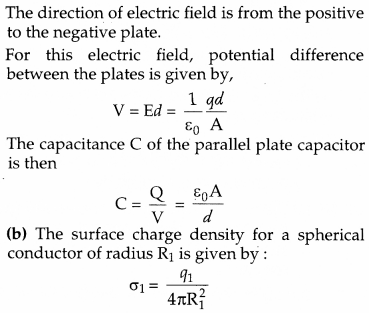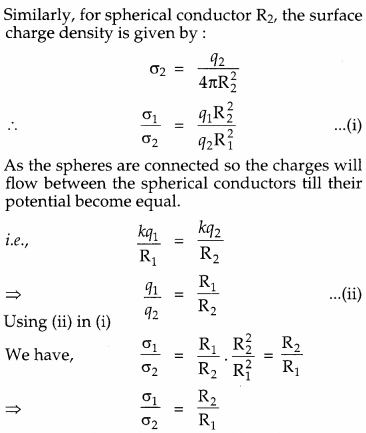Question 25.
(a) State Ampere’s circuital law, expressing it in the integral form.
(b) Two long coaxial insulated solenoids, S1 and S2 of equal lengths are wound one over the other as shown in the figure. A steady current “I” flow through the inner solenoid S1 to the other end B, which is connected to the outer solenoid S2 through which the same current “I” flows in the opposite direction so as to come out at end A. If n1 and n2 are the number of turns per unit length, find the magnitude and direction of the net magnetic field at a point
(i) inside on the axis and
(ii) outside the combined system.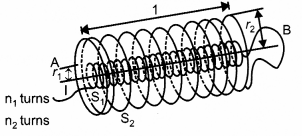(a) Ampere’s circuital law states that the circulation of the resultant magnetic field along a closed, plane curve is equal to µ0 times the total current crossing the area bounded by the closed curve, provided the electric field inside the loop remains constant.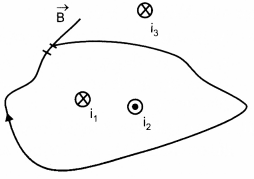In the above illustration, the Ampere’s circuital law can be written as follows :
$$\begin{array}{c}{\oint \overrightarrow{\mathrm{B}} \cdot \overrightarrow{d l}=\mu_{0} i} \\ {\text { where } i=i_{1}-i_{2}}\end{array}$$

(b) (i) The magnetic field due to a current carrying solenoid:
$$B=\mu_{0} n i$$

where, n = number of turns per unit length,
i = current through the solenoid.

Now, the magnetic field due to solenoid S1 will be in the upward direction and the magnetic field due to S2 will be in the downward direction (by right-hand screw rule).
\begin{aligned} \mathrm{B}_{\text {net}} &=\mathrm{B}_{1}-\mathrm{B}_{2} \\ \mathrm{B}_{\text {net}} &=\mu_{0} n_{1} \mathrm{I}-\mu_{0} n_{2} \mathrm{I} \\ &=\mu_{0} \mathrm{I}\left(n_{1}-n_{2}\right) \end{aligned}
(ii) The magnetic field is zero outside a solenoid.

Question 26.
(a) Name the e.m. waves which are suitable for radar systems used in aircraft navigation. Write the range of frequency of these waves.
(b) If the earth did not have atmosphere, would its average surface temperature be higher or lower than what it is now ? Explain.
(c) An e.m. wave exerts pressure on the surface on which it is incident. Justify.
(a) Microwaves are suitable for radar systems used in aircraft’s navigation. The range of frequency for these waves is 109 Hz to 1012 Hz.
(b) In the absence of atmosphere, there would be no greenhouse effect on the surface of the Earth. As a result, the temperature of the Earth would decrease rapidly, making it difficult for human survival.
(c) The momentum transported by electromagnetic waves is given by
$$p=\frac{\mathrm{U}}{c}$$

where U is the energy transported by electromagnetic waves in a given time and c is speed of electromagnetic waves in free space. As a result, when these waves strike a surface, pressure and hence force is exerted by them on the surface.

Question 27.
(a) Deduce the expression, N = No e-λt, for the law of radioactive decay.
(b) (i) Write symbolically the process expressing the β+ decay of $$\overset { 22 }{ 11 }$$Na. Also write the basic nuclear process underlying this decay.
(ii) Is the nucleus formed in the decay of the nucleus $$\overset { 22 }{ 11 }$$Na, an isotope or isobar? 
(a) Suppose initially the number of atoms in radioactive element is N0 and N the number of atoms after time t. After time t, let dN be the number of atoms which disintegrate in a short interval dt,

then rate of disintegration will be, dN/dt, this is also called the activity of the element.

According to Rutherford-Soddy law,
$$\begin{array}{l}{\frac{d \mathbf{N}}{d t} \propto \mathbf{N}} \\ {\frac{d \mathbf{N}}{d t}=-\lambda \mathbf{N}}\end{array}$$ ……… (i)

where λ is a constant, called decay constant or disintegration constant of the element. Its unit is s-1. Negative sign shows that the rate of disintegration decreases with increase of time. For a given element λ, is a constant and is different for different elements. Equation (i) may be rewritten asThe neutron-proton ratio is also an important factor that determines the stability of a nucleus. A proton-rich nucleus undergoes positron emission (β+ decay), so as to improve the neutron- proton ratio. The positron emission decreases proton number relative to neutron number and the product nucleus becomes relatively more stable. In β+ decay, neutrino (v) is emitted so as to conserve spin.
ii) The nucleus so formed is an isobar of $$11N\overset { 22 }{ a }$$ because the mass number is same, but the atomic numbers are different.

Question 28.
(a) (i) ‘Two independent mono-chromatic sources of light cannot produce a sustained interference pattern’. Give reason.
(ii) Light wave each of amplitude ‘a’ and frequency ‘ω’, emanating from two coherent light sources superpose at a point. If the displacements due to these waves is given by y1 = a cos ωt and y2 = a cos (ωt + Φ) where Φ is the phase difference between the two, obtain the expression for the resultant intensity at the point.
(b) In Young’s double slit experiment, using monochromatic light of wavelength λ, the intensity of light at a point on the screen where path difference is λ, is K units. Find out the intensity of light at a point where path difference is λ/3. 
OR
(a) How does one demonstrate, using a suitable diagram, that unpolarised light when passed through a Polaroid gets polarized ?
(b) A beam of unpolarised light is incident on a glass-air interface. Show, using a suitable ray diagram, that light reflected from the interface is totally polarized, when µ = tan iB where µ is the refractive index of glass with respect to air and iB is the Brewster’s angle.
(a) (i) The condition for the sustained interference is that both the sources must be coherent (i.e., they must have the same wavelength and the same frequency, and they must have the same phase or constant phase difference).

Two sources are monochromatic if they have the same frequency and wavelength. Since they are independent, i.e., they have different phases with irregular difference, they are not coherent sources.
(ii)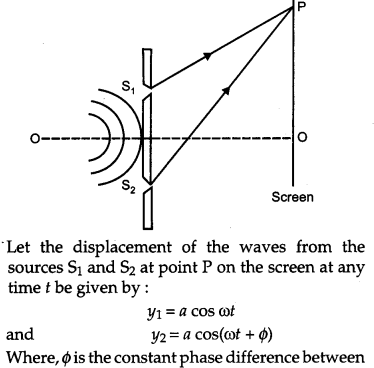The phenomenon of restricting the vibration of light (electric vector) in a particular direction perpendicular to the direction of the wave propagation is called polarization of light.

When unpolarised light is passed through a Polaroid, only those vibrations of light pass through the crystal, which are parallel to the axis of the crystal (AB). All other vibrations are absorbed and that is why intensity of the emerging light is reduced.

The plane ABCD in which the vibrations of the polarized light are confined is called the plane of vibration. The plane KLMN that is perpendicular to the plane of vibration is defined as the plane of polarization.

(b) When unpolarised light is incident on the glass-air interference at Brewster angle Z’B, then reflected light is totally polarised. This is called Brewster’s Law.

When light is incident at Brewster angle, the reflected component OB and the refracted component OC are mutually perpendicular to each Other.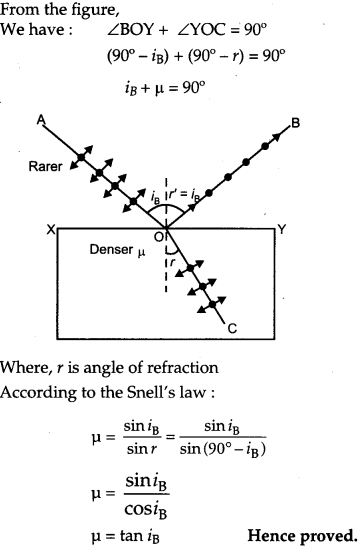Question 29.
(a) Describe a simple experiment (or activity) to show that the polarity of emf induced in a coil is always such that it tends to produce a current which opposes the change of magnetic flux that produces it.
(b) The current flowing through an inductor of self inductance L is continuously increasing. Plot a graph showing the variation.
(i) Magnetic flux versus the current
(ii) Induced emf versus dl/dt
(iii) Magnetic potential energy stored versus the current. 
OR
(a) Draw a schematic sketch of an ac generator describing its basic elements. State briefly its working principle. Show a plot of variation of
(i) Magnetic flux and
(ii) Alternating emf versus time generated by a loop of wire rotating in a magnetic field.
(b) Why is choke coil needed in the use of fluorescent tubes with ac mains ?
(a) Lenz’s law : According to Lenz’s law, the polarity of the induced emf is such that it opposes a change in magnetic flux responsible for its production.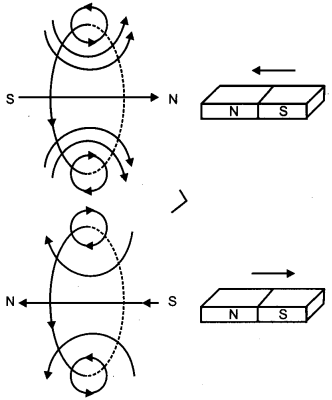When the north pole of a bar magnet is pushed towards the coil, the amount of magnetic flux linked with the coil increase. Current is reduced in the coil from a direction such that it opposes the increase in magnetic flux. This is possible only when the current induced in the coil is in anti-clockwise direction, with respect to an observer. The magnetic moment M associated with this induced emf has north polarity, towards the north pole of the approaching bar magnet.

Similarly, when the north pole of the bar magnet is moved away from the coil, the magnetic flux linked with the coil decreases. To counter this decrease in magnetic flux, current is induced in the coil in clockwise direction so that its south pole faces the receding north pole of the bar magnet. This would result in an attractive force which opposes the motion of the magnet and the corresponding decrease in magnetic flux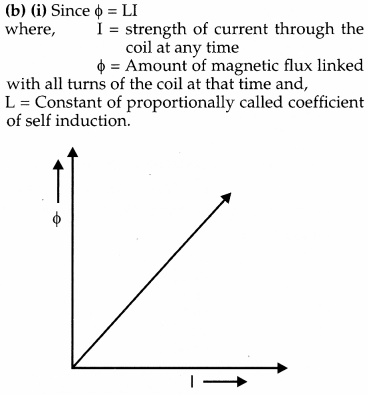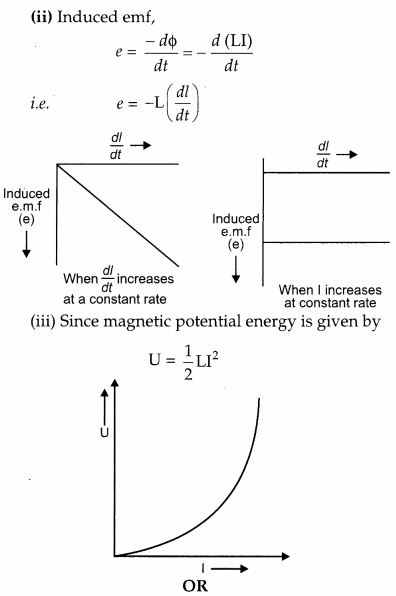It works on the process of electromagnetic induction, i.e., when a coil rotates continuously in a magnetic field, the effective area of the coil, linked (normally) with the magnetic field lines, changes continuously with time. This variation of magnetic flux with time results in the production of a (alternating) emf in the coil.(b) A choke coil is an electrical appliance used for controlling current in an a.c. circuit. Therefore, if we use a resistance R for the same purpose, a lot of energy would be wasted in the form of heat etc.

Question 30.
(a) State briefly the processes involved in the formation of p-n junction explaining clearly how the depletion region is formed.
(b) Using the necessary circuit diagram, show how the V-I characteristics of a p-n junction are obtained in
(i) Forward biasing
(ii) Reverse biasing
How are these characteristics made use of in rectification ? 
OR
(a) Differentiate between three segments of a transistor on the basis of their size and level of doping.
(b) How is a transistor biased to be in active state ?
(c) With the help of necessary circuit diagram, describe briefly how n-p-n transistor in CE configuration amplifies a small sinusoidal input voltage. Write the expression for the ac current gain.
(a) As we know that n-type semi-conductor has more concentration of electrons than that of a hole and p-type semi-conductor has more concentration of holes than an electron. Due to the difference in concentration of charge carriers in the two regions of p-n junction, the holes diffuse from p-side to //-side and electrons diffuse from n-side to p-side.

When an electron diffuses from n to p, it leaves behind an ionized donor on n-side. The ionized donor (+ve charge) is immobile as it is bounded by the surrounding atoms. Therefore, a layer of positive charge is developed on the n-side of the junction. Similarly, a layer of negative charge is developed on the p-side.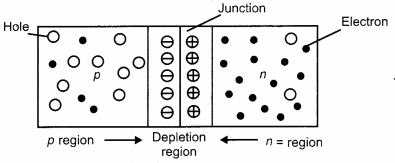Hence, a space-charge region is formed on both side of the junction, which has immobile ions and is devoid of any charge carrier, called as depletion layer or depletion region.
(b)Using the circuit arrangements shown in fig (a) and fig (b), we study the variation of current with applied voltage to obtain the V-I characteristics shown below.From the V-I characteristics of a junction diode, it is clear that it allows the current to pass only when it is forward biased. So when an alternating voltage is applied across the diode, current flows only during that part of the cycle when it is forward biased.

### CBSE Previous Year Question Papers Class 12 Physics 2014 Delhi Set-II

Note : Except for the following questions, all the remaining questions have been asked in previous Set.

Question 1.
Define the term ‘electrical conductivity’ of a metallic wire. Write its S.I. unit. 
The electrical conductivity of a metallic wire is defined as the ratio of the current density to the electric field it creates.
Electrical conductivity, $$\sigma=\frac{\mathrm{J}}{\mathrm{E}}$$
S.I. unit = (ohm m)-1
OR
The reciprocal of resistivity of a material is called its electrical conductivity.
OR  $$\begin{array}{l}{\text { Conductivity }=\frac{1}{\text { Resistivity }}} \\ {\qquad \sigma=\frac{1}{\rho}}\end{array}$$

Question 2.
The carrier wave is represented by C (t) = 5 sin (10 πt) volt A modulating signal is a square wave as shown. Determine modulation index.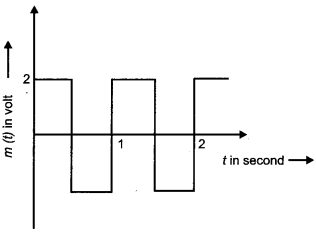Question 3.
An electric dipole of length 2 cm, when placed with its axis making an angle of 60° with a uniform electric field, experiences a torque of 8√3 Nm. Calculate the potential energy of the dipole, if it has a charge of ±4 nC.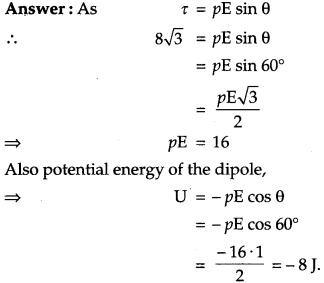Question 4.
A proton and an alpha particle are accelerated through the same potential ? Which one of the two has
(i) greater value of de-Broglie wavelength associated with it and
(i) de-Broglie wavelength of a particle depends upon its mass and charge for same accelerating potential such that
$$\lambda \propto \frac{1}{\sqrt{(\text { Mass })(\text { Charge })}}$$

If mass and charge of a proton are mp and e respectively, then, mass and charge of an alpha particle are 4mp and 2e respectively. Where, e is the charge of an electron
\begin{aligned} \frac{\lambda_{p}}{\lambda_{\alpha}} &=\sqrt{\frac{m_{\alpha} q_{\alpha}}{m_{p} q_{p}}} \\ &=\sqrt{\frac{\left(4 m_{p}\right)(2 e)}{\left(m_{p}\right)(e)}}=2 \sqrt{2} \end{aligned}

Thus, de-Broglie wavelength associated with proton is 2√2 times of the de-Broglie wavelength of alpha particle.
(ii) K.E. ∝ q (for same accelerating potential).
Charge of an alpha particle is more as compared to a proton. So, it will have a greater value of K.E. Hence, proton will have lesser kinetic energy.

Question 5.
Given a uniform electric field $$\overrightarrow{\mathbf{E}}=2 \times 10^{3} \hat{i} \mathrm{N} / \mathrm{C}$$ Find the flux of this field through a square of side 20 cm, whose plane is parallel to the Y-Z plane. What would be the flux through the same square, if the plane makes an angle of 30° with the X-axis ? 
When the plan is parallel to the Y-Z plane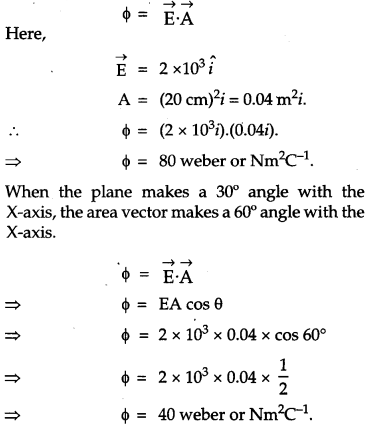Question 6.
A 12.9 eV beam of electronic is used to bombard gaseous hydrogen at room temperature. Up to which energy level the hydrogen atoms would be excited ? Calculate the wavelength of the first member of Paschen series and first member of Balmer series. 
Energy of the electron in the nth state of an atom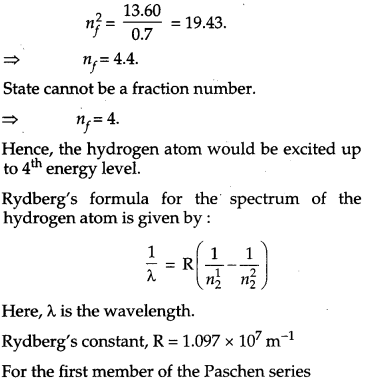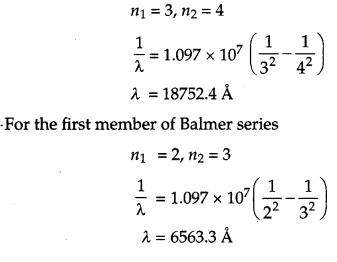Question 7.
(a) Name the e.m. waves which are used for the treatment of certain forms of cancer. Write their frequency range.
(b) Thin ozone layer on top of stratosphere is crucial for human survival. Why ?
(c) Why is the amount of the momentum transferred by the e.m. waves incident on the surface so small ?

(a) Gamma rays are used for the treatment of certain forms of cancer. Their frequency range is 3 x 1019 Hz to 5 x 1020 Hz.
(b) The thin ozone layer on top of stratosphere absorb most of the harmful ultraviolet rays coming from the Sun towards the Earth. They include UVA, UVB and UVC radiations, which can destroy the life system on the Earth. Hence, this layer is crucial for human survival.
(c) The momentum transported by electroma-gnetic waves is given byQuestion 8.
A potentiometer wire of length 1.0 m has a resistance of 15 Ω. It is connected to a 5 V battery in series with a resistance of 5 Ω. Determine the emf of the primary cell which gives a balance point at 60 cm. 
Current through potentiometer wire,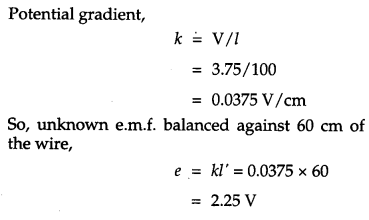### CBSE Previous Year Question Papers Class 12 Physics 2014 Delhi Set-III

Note : Except for the following questions, all the remaining questions have been asked in previous Sets.

Question 1.
Define the term ‘drift velocity’ of charge carriers in a conductor and write its relationship with the current flowing through it. 
The net speed achieved by an electron in a current carrying conductor is called as drift velocity. The average velocity acquired by the free electrons along the length of a metallic conductor under a potential difference applied across the conductor is called drift velocity of the electrons.
$$v_{d}=\frac{\mathbf{I}}{n e \mathbf{A}}$$
Here:
I is the current flowing through the conductor.
n is the number density of an electron.
A is the area of the conductor,
e is the charge of the electron.

Question 2.
The carrier wave of a signal is given by C(t) = 3 sin (8πt) volt. The modulating signal is a square wave as shown. Find its modulation index.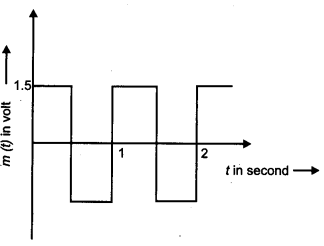Question 3.
Plot a graph showing variation of current versus voltage for the material Ga. 
Current-Voltage characteristics graph for Ga:Question 4.
An electric dipole of length 2 cm, when placed with its axis making an angle of 60° with a uniform electric field, experiences a torque of 6√3 Nm. Calculate the potential energy of the dipole, if it has a charge of ±2 nC. 
AsQuestion 5.
A deuteron and an alpha particle are accelerated with the same accelerating potential. 
Which one of the two has
(1) greater value of de-Broglie wavelength, associated with it, and
(2) less kinetic energy ? Explain.
(1) de-Broglie wavelength of a particle is dependent on its mass and charge for same accelerating potential, such that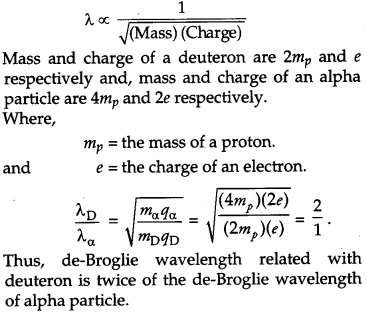(2) K.E. ∝q
(for same accelerating potential)
Charge of a deuteron is less as compared to an alpha particle. So, deuteron will have less kinetic energy.

Question 6.
Given a uniform electric field $$\overrightarrow{\mathrm{E}}=4 \times 10^{3} \hat{i} \mathrm{N} / \mathrm{C}$$. Find the flux of this field through a square of 5 cm on a side whose plane is parallel to the Y-Z plane. What would be the flux through the same square, if the plane makes an angle of 30° with the X-axis ? 
When the plane is parallel to the Y-Z plane: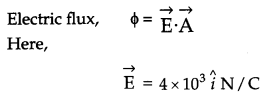Question 7.
A 12.3 eV electron beam is used to bombard gaseous hydrogen at room temperature. Upto which energy level the hydrogen atoms would be excited ? Calculate the wavelength of the second member of Lyman series and second member of Balmer series. 
Let the hydrogen atoms be excited to nth energy level.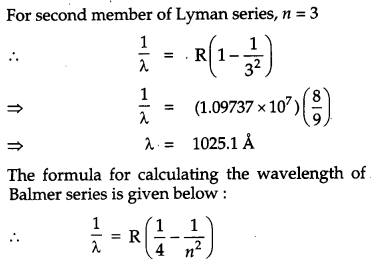Question 8.